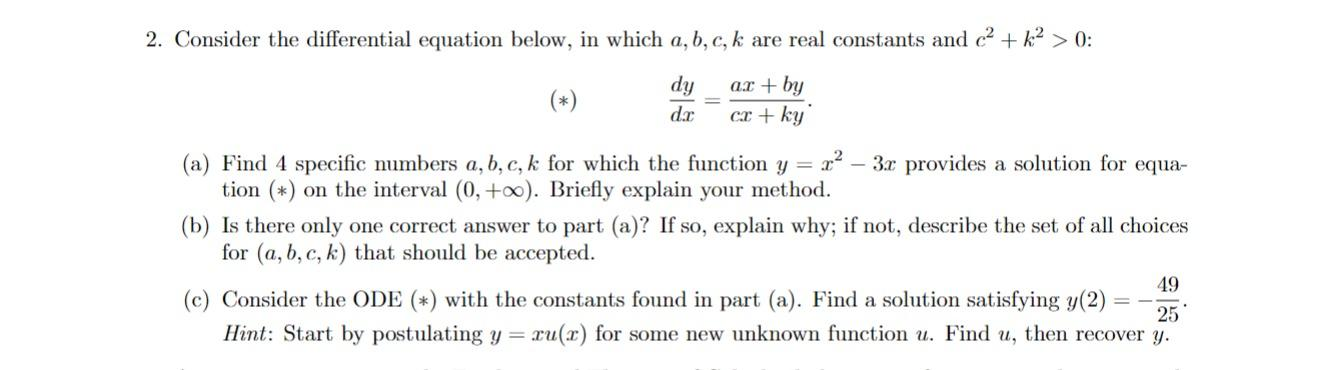# Question 2. Consider the differential equation below, in which $$a, b, c, k$$ are real constants and $$c^{2}+k^{2}>0$$ : $\frac{d y}{d x}=\frac{a x+b y}{c x+k y}$ (a) Find 4 specific numbers $$a, b, c, k$$ for which the function $$y=x^{2}-3 x$$ provides a solution for equation $$(*)$$ on the interval $$(0,+\infty)$$. Briefly explain your method. (b) Is there only one correct answer to part (a)? If so, explain why; if not, describe the set of all choices for $$(a, b, c, k)$$ that should be accepted. (c) Consider the ODE (*) with the constants found in part (a). Find a solution satisfying $$y(2)=-\frac{49}{25}$$. Hint: Start by postulating $$y=x u(x)$$ for some new unknown function $$u$$. Find $$u$$, then recover $$y$$.Transcribed Image Text: 2. Consider the differential equation below, in which $$a, b, c, k$$ are real constants and $$c^{2}+k^{2}>0$$ : $\frac{d y}{d x}=\frac{a x+b y}{c x+k y}$ (a) Find 4 specific numbers $$a, b, c, k$$ for which the function $$y=x^{2}-3 x$$ provides a solution for equation $$(*)$$ on the interval $$(0,+\infty)$$. Briefly explain your method. (b) Is there only one correct answer to part (a)? If so, explain why; if not, describe the set of all choices for $$(a, b, c, k)$$ that should be accepted. (c) Consider the ODE (*) with the constants found in part (a). Find a solution satisfying $$y(2)=-\frac{49}{25}$$. Hint: Start by postulating $$y=x u(x)$$ for some new unknown function $$u$$. Find $$u$$, then recover $$y$$.
Transcribed Image Text: 2. Consider the differential equation below, in which $$a, b, c, k$$ are real constants and $$c^{2}+k^{2}>0$$ : $\frac{d y}{d x}=\frac{a x+b y}{c x+k y}$ (a) Find 4 specific numbers $$a, b, c, k$$ for which the function $$y=x^{2}-3 x$$ provides a solution for equation $$(*)$$ on the interval $$(0,+\infty)$$. Briefly explain your method. (b) Is there only one correct answer to part (a)? If so, explain why; if not, describe the set of all choices for $$(a, b, c, k)$$ that should be accepted. (c) Consider the ODE (*) with the constants found in part (a). Find a solution satisfying $$y(2)=-\frac{49}{25}$$. Hint: Start by postulating $$y=x u(x)$$ for some new unknown function $$u$$. Find $$u$$, then recover $$y$$.&#12304;General guidance&#12305;The answer provided below has been developed in a clear step by step manner.Step1/3(a)To find values of a, b, c, and k for which the function$$\mathrm{{y}={x}^{{2}}-{3}{x}}$$ is a solution to the given differential equation on the interval (0, +&#8734;), Let us differentiate y with respect to x $$\mathrm{{y}={x}^{{2}}-{3}{x},}$$ $$\mathrm{{y}'={2}{x}-{3}}$$Now substitute it into the given differential equation. We can for the values of a, b, c, and k On substituting the above equation into the given differential equation, we obtain as below$$\mathrm{{\left({2}{x}-{3}\right)}=\frac{{{a}{x}+{b}{\left({x}^{{2}}-{3}{x}\right)}}}{{{c}{x}+{k}{\left({x}^{{2}}-{3}{x}\right)}}}}$$Multiply both the sides by $$\mathrm{{c}{x}+{k}{\left({x}^{{2}}-{3}{x}\right)}}$$$$\mathrm{{\left({2}{x}-{3}\right)}{\left({c}{x}+{k}{\left({x}^{{2}}-{3}{x}\right)}\right)}={a}{x}{\left({c}{x}+{k}{\left({x}^{{2}}-{3}{x}\right)}\right)}+{b}{\left({x}^{{2}}-{3}{x}\right)}{\left({c}{x}+{k}{\left({x}^{{2}}-{3}{x}\right)}\right)}}$$Equation the similar terms on both the sides we get$$\mathrm{{\left({2}{c}-{9}{k}\right)}{x}^{{3}}+{\left({k}{c}-{6}{b}\right)}{x}^{{2}}+{\left({a}{k}-{3}{c}\right)}{x}+{b}{k}={0}}$$The above equation is true for all the values of x in the interval (0, +&#8734;) and hence the coefficients of all the powers of x will be zero. Explanation:Please refer to solution in this step.Step2/3We get these equations$$\mathrm{{2}{c}-{9}{k}={0}}$$$$\mathrm{{k}{c}-{6}{b}={0}}$$$$\mathrm{{a}{k}-{3}{c}={0}}$$$$\mathrm{{b}{k}={0}}$$From the fourth equation it ... See the full answer